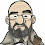## Monday, December 3, 2012

### NFL Picks Contest- Results and Standings

Here are the results and updated standings in the NFL Picks Contest

lightning- Houston (W 24-10) = 24 pts.
TwoMinuteWarning- Green Bay (W 23-14) = 19 pts.
Grange95-  Houston (W 24-10) = 24 pts.
ohcowboy12go- Houston (W 24-10) = 24 pts.
M Prosk- no pick = 0 pts.
Full House- New England (W 23-16) = 17 pts.
Music City Momma- Houston (W 24-10) = 24 pts.
Coach-  New England (W 23-16) = 17 pts.
Neophyte- no pick = 0 pts.
Waffles- Houston (W24-10) = 24 pts.
MOJO-  Green Bay (W 24-10) = 24 pts.
ggrouchie- New England (W23-16) = 17 pts.
Vegas Vic- Dallas (W 38-33) = 15 pts.
Captain Crunch- Houston (W 24-10) = 24 pts.

Here are the current standings;

grrouchie - 14+48+20+5+16+40+17 = 160 pts
MOJO - 40+19+22+13+16+18+24 = 152
Grange95 - 20+19+22+13+14+40+24 = 152
Coach - 20+19+22+13+16+40+17 = 147
Vegas Vic - 16+19+22+13+16+40+15 = 141
Music City Momma - 17+19+24+5+17+34+24 = 140
TwoMinuteWarning - 13+19+22+16+31+18+19 = 138
Captain Crunch - 13+11+18+16+31+18+24 = 131
lightning - 13+19+24+13+16+18+24 = 127
Waffles - 15+11+22+16+14+0+24 =102
Neophyte - 17+19+0+13+32+18+0 = 99
ohcowboy12go - 13+19+22+5+13+24=96
M Prosk - 17+0+41+5+17+0+0 = 80
Full House - 20+19+22-(34)+16+18+17 =78

Congratulations to grrouchie for holding on to the lead!

#### 1 comment:

1.There's a bad MoJo on the rise.
And Grange (who I somehow failed to meet/introduce myself to at the wpbt) is hot on my heels as well.

We need to cattle prod Prosk though - wtf man, wtf.